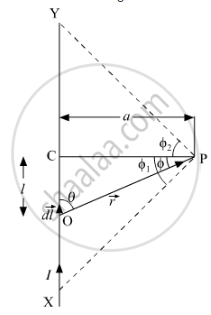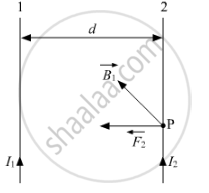# Two Infinitely Long Straight Parallel Wires, '1' and '2', Carrying Steady Currents I1 and I2 in the Same Direction Are Separated by a Distance D. Obtain the Expression for the Magnetic Field BDue to the Wire '1' Acting on Wire '2'. Hence Find Out, with the Help of a Suitable Diagram, - Physics

Two infinitely long straight parallel wires, '1' and '2', carrying steady currents I1 and I2 in the same direction are separated by a distance d. Obtain the expression for the magnetic field vecBdue to the wire '1' acting on wire '2'. Hence find out, with the help of a suitable diagram, the magnitude and direction of this force per unit length on wire '2' due to wire '1'. How does the nature of this force changes if the currents are in opposite direction? Use this expression to define the S.I. unit of current.

#### Solution

Consider a straight conductor XY lying in the plane of paper. Consider a point P at a perpendicular distance a from straight conductor.Magnetic field induction (B) at a point P due to current I passing through conductor XY is given by

B=(μ0I)/(4πa)[sinϕ_1+sinϕ_2]

where ϕ1 and ϕ2  are the angles made by point X and Y, respectively

At the centre of the infinite long wire, ϕ1=ϕ2=90°

B=(μ_0I)/(4πa)[sin90^@+sin^@]

⇒B=μ_0/(4π) (2I)/a   .....(1)Let 1 and 2 be two long infinite straight conductors. Let I1 and I2 be the current flowing through the conductor 1 and 2 and they are d distance apart from each other as shown in the figure.

The magnetic field induction (B) at a point P on conductor 2 due to current I1 passing through conductor 1 is given by

B=(μ_02I_1)/(4πd)   [From (1), where a=d]

According to right hand rule, the direction of this magnetic field is perpendicular to the plane of the paper inward.
Since the conductor 2 lies in this magnetic field of conductor 1, force experienced (F2) by unit length of conductor 2 will be

F2=B1I2×1=B1I2

∴ F2=μ_0/(4π) (2I_1I_2)/d

Conductor 1 also experiences the same amount of force, directed towards the conductor 2. Hence, conductor 1 and conductor 2 attract each other. Thus, two linear parallel conductors carrying currents in the same direction attract and repel each other, when the current flows in the opposite direction.

Let I_1=I_1=1A; r=1 m
Then,

F_1=F_2=F=10^(−7) (2xx1xx1)/1

⇒F=2×10^(−7)  N/m

Thus, one ampere is that value of constant current which when flowing through each of the two parallel uniform long linear conductors placed in free space at a distance of 1 m from each other will attract or repel each other with a force of 2 × 10−7 N per metre of their length.

Concept: Force Between Two Parallel Currents, the Ampere
Is there an error in this question or solution?
2014-2015 (March) Patna Set 2

Share